GRE Subject Test: Math : Finite, Infinite, Countable, Not Countable Classifications

Example Questions

Example Question #6 : Real Analysis

Which of the following sets is not an infinite set??

All numbersbetweenand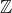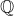All whole numbers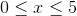All whole numbersExplanation:

Step 1: Determine the difference between Infinite and Finite Sets...

Finite Sets: A set that has very limited elements in it
Infinite Sets: Any set where I can always find another number between two given boundaries.

The Set of integers and rational numbers are infinite sets..
All numbers betweenandis also an infinite set because I can come up with infinite decimal numbers..

The set of whole numbers betweenandinclusive is a finite set because I ask for a specific type of number.. a whole number. Whole numbers cannot be decimals..

Example Question #1 : Real Analysis

Which of the following is a finite set?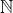All whole numbers betweenand, inclusive

All real numbers betweenand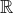All whole numbers betweenand, inclusive
Note: The set of real numbers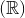, natural numbers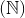are both infinite sets.
Step 1: The set of real numbers between two numbers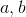is also an infinite set.
Step 2: The set of whole numbers betweenandinclusive is finite because there are only three numbers that are represented by the set. These numbers are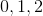.